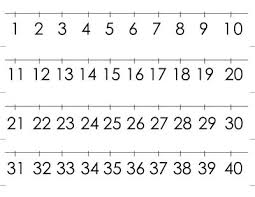# Numbers 13691

Write the number 126 as the sum of three numbers, which ratio is 2: 5: 7

a =  18
b =  45
c =  63

### Step-by-step explanation:Did you find an error or inaccuracy? Feel free to write us. Thank you!

Tips for related online calculators
Check out our ratio calculator.
Do you have a linear equation or system of equations and looking for its solution? Or do you have a quadratic equation?